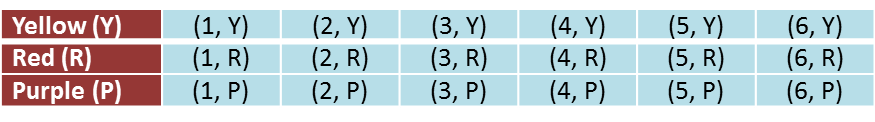# 7.5 Probability (II), SPM Practice (Long Questions)

Question 5:
A fair dice is tossed. Then a card is picked at random from a box containing a yellow, a red and a purple card.
(a) By using the letter Y to represent the yellow card, the letter R to represent the red card and the letter P to represent the purple card, complete the sample space in the diagram in the answer space.
(b) By listing down all the possible outcomes of the event, find the probability that
(i) a number less than six and a red card are chosen.
(ii) a number greater than three or a purple card is chosen.

(a)Solution:
(a)(b)

Question 6:
A coin is tossed and a dice is rolled simultaneously. By listing the sample of all the possible outcomes of the event, find the probability that
(a) a head and an odd number are obtained.
(b) a head or a number greater than 4 are obtained.

Solution: